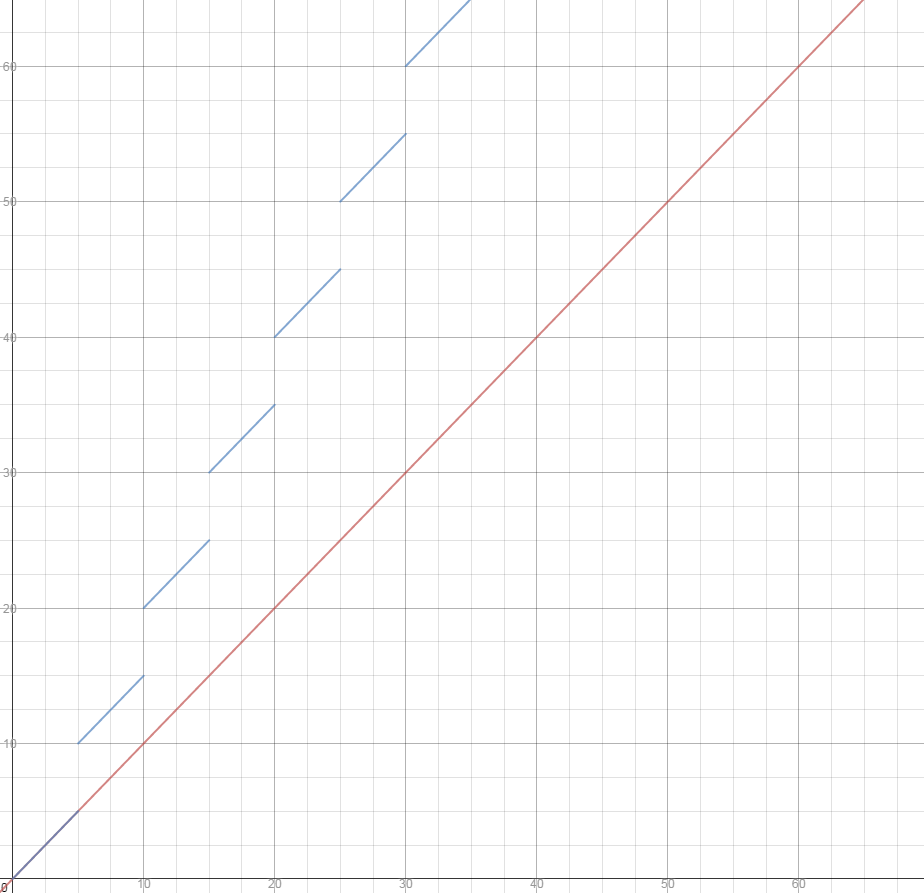# Long Question:

## Overview:

While I understand that over certain intervals (i.e. less than the width of the step), they may appear not to be because the step is outside of consideration, and over other intervals (much, much larger) the step seems continuous as opposed to discrete, but at the end of the day aren't these merely artifacts of the analysis, as opposed to properties of the underlying function?

Admittedly, I was never much good with the calculus of discrete functions, but I remember there being some substantial differences between their derivatives (e.g. the need to invoke the finite difference method as opposed to a limit).

Further, given the potential steepness of the step, and its unknown location, isn't predicting difficult?

## A Picture:

For example, the graph below shows a cost function for $n$ units with and without a step.

The red line is:

$$C(n)=5n$$

and the blue line is $$C(n) = n+(n-\mod (n,5))$$Analyzed over $(0,5)$ these would coincide, and an analyst might not have any expectation that increasing by a small factor would dramatically increase cost, and while the (simple) derivative at any point on the line is the same for either line (inside the step interval), this is very misleading.

Further, I chose $5$ at random, a more generalized idea might be something along the lines of:

$$C(n) = n+\frac{S(n-\mod(n,w))}{w}$$

where $S$ is the step cost, and $w$ is the width.

## Summary:

Given these concepts, why are step cost functions generally treated as less preferential in discussing models? Or is that just my experience, and not indicative of the way the field treats cost functions as a whole?

I ask this, because whenever I see a discussion of a cost function, it seems to be continuous, and whenever I see mention of a step function it seems to be an afterthought.

• Because it makes the maths easier. Generally, also output is not continuous either ;) Dec 4, 2014 at 21:39
• I think it's less relevant in the aggregate. Also, I don't think the analysis changes much either. Dec 4, 2014 at 22:21
• @FooBar, that's what I'm kind of getting at. The shortcut seems to leave a lot to be desired, given the prevalence of continuous functions as stand ins and the application of analytical methods that do not apply to discrete functions. Dec 5, 2014 at 2:32